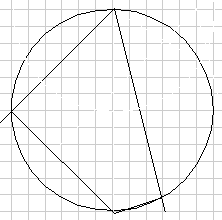ireallymetal
If you have a quadrilateral whose angles measure 90, 90, 60, 120 where the right angles are opposite each other does that mean that the quadrilateral is tangential. If it is can somebody show me how they arrived at that conclusion?

Homework Helper
Your question doesn't make any sense with the standard meaning of "tangential". Maybe you used the wrong word, or you forgot to tell us what the quadrilateral is tangential to.

If you explain what you think the question meant, somebody will probably be able to help.

d01phi

The quad with angles 90°, 60°, 90°, 120° is obviously symmetric, and it is well known that symmetric quads are tangential. Is that enough of a proof?

Otherwise I'd do a proof by construction: construct the angle bisectors at the 90° corners and use their intersection M as tangential circle center. Draw a perpendicular from one of the quad sides through M to find a point on the circle perimeter. There you go...

Homework Helper

OK, I guess this has come into use since I learned my geometry!

The quad with angles 90°, 60°, 90°, 120° is obviously symmetric

Draw a circle. Draw a diameter. On one side of the diameter, draw two lines at 45 degrees, so you have a 45-90-45 triangle with each vertex on the circle.

Use those points as 3 vertices of the quadilateral and draw angles at 60 and 120 intersecting at the 4th vertex. Since the 4th vertex angle must be 90, it also lies on the circle (because the angle in a semicircle is a right angle).

Is that a symmetrical quadrilateral? I wouldn't call it symmetrical.
(Apologies if this is another use of terminology that I'm not familar with - and also for the scrappy diagram.)There must be some other condition for the OP's statement to be true. Four angles do not fix the shape of a quadrilateral. In the diagram, you can draw any lines parallel to the sides and get different shaped quadrilaterals with the same angles. They can't all be tangential quadrilaterals.

d01phi